# Voltage Divider Circuits

## DC Electric Circuits

• #### Question 1

 Don’t just sit there! Build something!!

Learning to mathematically analyze circuits requires much study and practice. Typically, students practice by working through lots of sample problems and checking their answers against those provided by the textbook or the instructor. While this is good, there is a much better way.

You will learn much more by actually building and analyzing real circuits, letting your test equipment provide the “answers” instead of a book or another person. For successful circuit-building exercises, follow these steps:

1. Carefully measure and record all component values prior to circuit construction.
2. Draw the schematic diagram for the circuit to be analyzed.
3. Carefully build this circuit on a breadboard or other convenient medium.
4. Check the accuracy of the circuit’s construction, following each wire to each connection point, and verifying these elements one-by-one on the diagram.
5. Mathematically analyze the circuit, solving for all values of voltage, current, etc.
6. Carefully measure those quantities, to verify the accuracy of your analysis.
7. If there are any substantial errors (greater than a few percent), carefully check your circuit’s construction against the diagram, then carefully re-calculate the values and re-measure.

Avoid very high and very low resistor values, to avoid measurement errors caused by meter “loading”. I recommend resistors between 1 kΩ and 100 kΩ, unless, of course, the purpose of the circuit is to illustrate the effects of meter loading!

One way you can save time and reduce the possibility of error is to begin with a very simple circuit and incrementally add components to increase its complexity after each analysis, rather than building a whole new circuit for each practice problem. Another time-saving technique is to re-use the same components in a variety of different circuit configurations. This way, you won’t have to measure any component’s value more than once.

• #### Question 2

Calculate the output voltages of these two voltage divider circuits (VA and VB):Now, calculate the voltage between points A (red lead) and B (black lead) (VAB).

• #### Question 3

We know that the current in a series circuit may be calculated with this formula:

 I = Etotal Rtotal

We also know that the voltage dropped across any single resistor in a series circuit may be calculated with this formula:

 ER = I R

Combine these two formulae into one, in such a way that the I variable is eliminated, leaving only ER expressed in terms of Etotal, Rtotal, and R.

• #### Question 4

Determine the amount of voltage dropped by each resistor in this circuit, if each resistor has a color code of Brn, Blk, Red, Gld (assume perfectly precise resistance values - 0% error):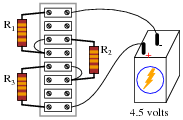Current through each resistor
Power dissipated by each resistor
Ratio of each resistor’s voltage drop to battery voltage ER/Ebat
Ratio of each resistor’s resistance to the total circuit resistance R/Rtotal
• #### Question 5

Calculate the voltage dropped by each of these resistors, given a battery voltage of 9 volts. The resistor color codes are as follows (assume 0% error on all resistor values):

R1 = Brn, Grn, Red, Gld
R2 = Yel, Vio, Org, Gld
R3 = Red, Grn, Red, Gld
R4 = Wht, Blk, Red, Gld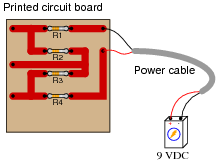Now, re-calculate all resistor voltage drops for a scenario where the total voltage is different: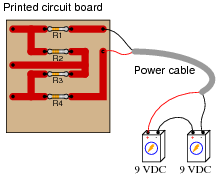• #### Question 6

Design a voltage divider circuit that splits the power supply voltage into the following percentages:• #### Question 7

Design a voltage divider circuit that splits the power supply voltage into the following percentages:• #### Question 8

Calculate VA (voltage at point A with respect to ground) and VB (voltage at point B with respect to ground) in the following circuit:Now, calculate the voltage between points A and B (VAB).

• #### Question 9

Many manufacturing processes are electrochemical in nature, meaning that electricity is used to promote or force chemical reactions to occur. One such industry is aluminum smelting, where large amounts of DC current (typically several hundred thousand amperes!) is used to turn alumina (Al2O3) into pure metallic aluminum (Al):The alumina/electrolyte mixture is a molten bath of chemicals, lighter than pure aluminum itself. Molecules of pure aluminum precipitate out of this mix and settle at the bottom of the “pot” where the molten metal is periodically pumped out for further refining and processing. Fresh alumina powder is periodically dropped into the top of the pot to replenish what is converted into aluminum metal.

Although the amount of current necessary to smelt aluminum in this manner is huge, the voltage drop across each pot is only about 4 volts. In order to keep the voltage and current levels reasonable in a large smelting facility, many of these pots are connected in series, where they act somewhat like resistors (being energy loads rather than energy sources):A typical “pot-line” might have 240 pots connected in series. With a voltage drop of just over 4 volts apiece, the total voltage powering this huge series circuit averages around 1000 volts DC:With this level of voltage in use, electrical safety is a serious consideration! To ensure the safety of personnel if they must perform work around a pot, the system is equipped with a “movable ground,” consisting of a large switch on wheels that may be connected to the steel frame of the shelter (with concrete pilings penetrating deep into the soil) and to the desired pot. Assuming a voltage drop of exactly 4.2 volts across each pot, note what effect the ground’s position has on the voltages around the circuit measured with respect to ground:Determine the voltages (with respect to earth ground) for each of the points (dots) in the following schematic diagram, for the ground location shown:• #### Question 10

Draw an equivalent schematic diagram for this circuit, then calculate the voltage dropped by each of these resistors, given a battery voltage of 9 volts. The resistor color codes are as follows (assume 0% error on all resistor values):

R1 = Brn, Grn, Red, Gld
R2 = Yel, Vio, Org, Gld
R3 = Red, Grn, Red, Gld
R4 = Wht, Blk, Red, Gld
R5 = Brn, Blk, Org, Gld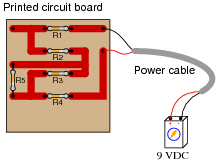Compare the voltage dropped across R1, R2, R3, and R4, with and without R5 in the circuit. What general conclusions may be drawn from these voltage figures?

• #### Question 11

The formula for calculating voltage across a resistor in a series circuit is as follows:

 VR = Vtotal ( R Rtotal )

In a simple-series circuit with one voltage source and three resistors, we may re-write this formula to be more specific:

 VR1 = Vsource ( R1 R1 + R2 + R3 )

Suppose we have such a series circuit with a source voltage of 15 volts, and resistor values of R1 = 1 kΩ and R2 = 8.1 kΩ. Algebraically manipulate this formula to solve for R3 in terms of all the other variables, then determine the necessary resistance value of R3 to obtain a 0.2 volt drop across resistor R1.

• #### Question 12

What will happen to the voltages across resistors R1 and R2 when the load is connected to the divider circuit?• #### Question 13

Calculate both the maximum and the minimum amount of voltage obtainable from this potentiometer circuit (as measured between the wiper and ground):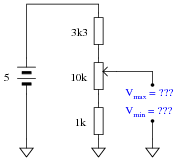• #### Question 14

Calculate both the maximum and the minimum amount of voltage that each of the voltmeters will register, at each of the potentiometer’s extreme positions:• #### Question 15

As adjustable devices, potentiometers may be set at a number of different positions. It is often helpful to express the position of a potentiometer’s wiper as a fraction of full travel: a number between 0 and 1, inclusive. Here are several pictorial examples of this, with the variable m designating this travel value (the choice of which alphabetical character to use for this variable is arbitrary):Using an algebraic variable to represent potentiometer position allows us to write equations describing the outputs of voltage divider circuits employing potentiometers. Note the following examples: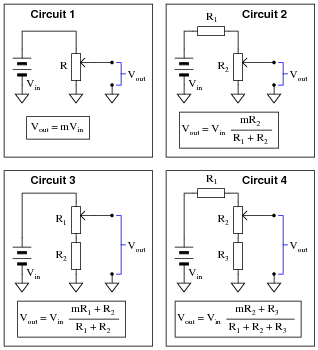Algebraically manipulate these four equations so as to solve for m in each case. This will yield equations telling you where to set each potentiometer to obtain a desired output voltage given the input voltage and all resistance values (m = …).

• #### Question 16

When the 5 kΩ potentiometer in this circuit is set to its 0%, 25%, 50%, 75%, and 100% positions, the following output voltages are obtained (measured with respect to ground, of course):At 0% setting, Vout = 0 V
At 25% setting, Vout = 2.5 V
At 50% setting, Vout = 5 V
At 75% setting, Vout = 7.5 V
At 100% setting, Vout = 10 V

Calculate what the output voltages will be if a 1 kΩ load resistor is connected between the “Vout” terminal and ground:At 0% setting, Vout =
At 25% setting, Vout =
At 50% setting, Vout =
At 75% setting, Vout =
At 100% setting, Vout =
• #### Question 17

Determine the voltages (with respect to ground) at points A and B in this circuit under four different conditions: both loads off, load 1 on (only), load 2 on (only), and both loads on:• #### Question 18

Calculate both the total resistance of this voltage divider circuit (as “seen” from the perspective of the 25 volt source) and its output voltage (as measured from the Vout terminal to ground):Note that all potentiometers in this circuit are set exactly to mid-position (50%, or m = 0.5).

• #### Question 19

Calculate both the total resistance of this voltage divider circuit (as ßeen” from the perspective of the 25 volt source) and its output voltage (as measured from the Vout terminal to ground):Note that the two 5 kΩ potentiometers are set to their 80% positions (m = 0.8), while the 100 kΩ potentiometer is set exactly to mid-position (50%, or m = 0.5).

• #### Question 20

Calculate both the total resistance of this voltage divider circuit (as ßeen” from the perspective of the 25 volt source) and its output voltage (as measured from the Vout terminal to ground):Note that the upper 5 kΩ potentiometer is set to its 20% position (m = 0.2), while the lower 5 kΩ potentiometer is set to its 90% position (m = 0.9), and the 100 kΩ potentiometer is set to its 40% position (m = 0.4).

• #### Question 21

Which voltage divider circuit will be least affected by the connection of identical loads? Explain your answer.What advantage does the other voltage divider have over the circuit that is least affected by the connection of a load?

• #### Question 22

A student builds the following voltage divider circuit so she can power a 6-volt lamp from a 15-volt power supply: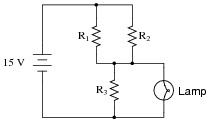When built, the circuit works just as it should. However, after operating successfully for hours, the lamp suddenly goes dark. Identify all the possible faults you can think of in this circuit which could account for the lamp not glowing anymore.

• #### Question 23

One of the resistors in this voltage divider circuit is failed open. Based on the voltage readings shown at each load, determine which one it is: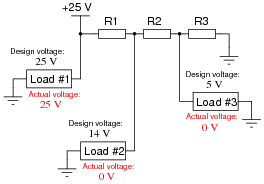• #### Question 24

One of the resistors in this voltage divider circuit is failed open. Based on the voltage readings shown at each load, determine which one it is: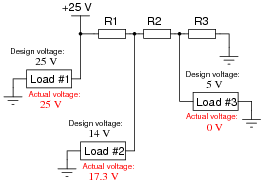• #### Question 25

One of the resistors in this voltage divider circuit is failed (either open or shorted). Based on the voltage readings shown at each load, determine which one and what type of failure it is: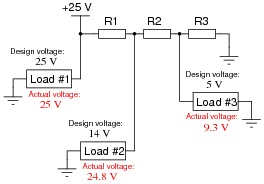• #### Question 26

One of the resistors in this voltage divider circuit has failed (either open or shorted). Based on the voltage readings shown at each load, comparing what each load voltage is versus what it should be, determine which resistor has failed and what type of failure it is: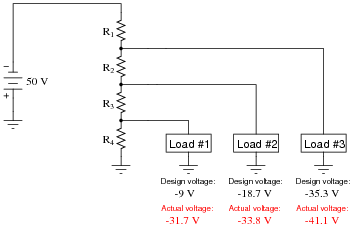• #### Question 27

One of the resistors in this voltage divider circuit has failed (either open or shorted). Based on the voltage readings shown at each load, comparing what each load voltage is versus what it should be, determine which resistor has failed and what type of failure it is: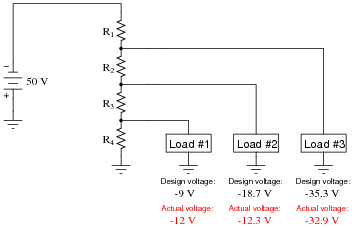• #### Question 28

One of the resistors in this voltage divider circuit has failed (either open or shorted). Based on the voltage readings shown at each load, comparing what each load voltage is versus what it should be, determine which resistor has failed and what type of failure it is: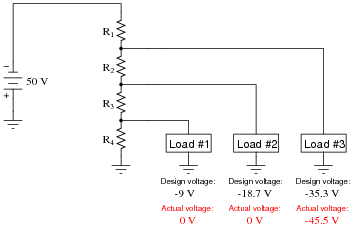• #### Question 29

One of the resistors in this voltage divider circuit has failed (either open or shorted). Based on the voltage readings shown at each load, comparing what each load voltage is versus what it should be, determine which resistor has failed and what type of failure it is: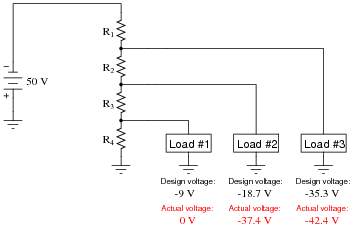• #### Question 30

Size the resistor in this voltage divider circuit to provide 3.2 volts to the load, assuming that the load will draw 10 mA of current at this voltage:As part of your design, include the power dissipation ratings of both resistors.

• #### Question 31

Size the resistor in this voltage divider circuit to provide 5 volts to the load, assuming that the load will draw 75 mA of current at this voltage:As part of your design, include the power dissipation ratings of both resistors.

• #### Question 32

Size both resistors in this voltage divider circuit to provide 6 volts to the load, assuming that the load will draw 7 mA of current at this voltage, and to have a “bleeder” current of 1 mA going through R2:As part of your design, include the power dissipation ratings of both resistors.

• #### Question 33

Explain what will happen to the first load’s voltage and current in this voltage divider circuit, as a second load is connected as shown: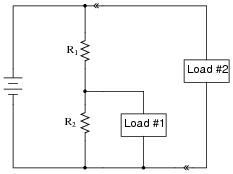• #### Question 34

Explain what will happen to the first load’s voltage and current in this voltage divider circuit if the second load develops a short-circuit fault:• #### Question 35

Size all three resistors in this voltage divider circuit to provide the necessary voltages to the loads, given the load voltage and current specifications shown:Assume a bleed current of 1.5 mA. As part of your design, include the power dissipation ratings of all resistors.

• #### Question 36

Old vacuum-tube based electronic circuits often required several different voltage levels for proper operation. An easy way to obtain these different power supply voltages was to take a single, high-voltage power supply circuit and “divide” the total voltage into smaller divisions.

These voltage divider circuits also made provision for a small amount of “wasted” current through the divider called a bleeder current, designed to discharge the high voltage output of the power supply quickly when it was turned off.

Design a high-voltage divider to provide the following loads with their necessary voltages, plus a “bleeder” current of 5 mA (the amount of current going through resistor R4):• #### Question 37

Suppose a voltmeter has a range of 0 to 10 volts, and an internal resistance of 100 kΩ: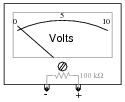Show how a single resistor could be connected to this voltmeter to extend its range to 0 to 50 volts. Calculate the resistance of this “range” resistor, as well as its necessary power dissipation rating.

### Related Content

Published under the terms and conditions of the Creative Commons Attribution License•JoshuaXD February 22, 2021

Plus signs are missing from these questions and answers, such as in question 11, where the denominator of the voltage divider equation appears as “R1 R2 R3” instead of “R1+R2+R3”. Same in the answer to question 15. Likely elsewhere as well, as Find shows no results for “+” anywhere on the page. I’m using Chrome 88.0.4324.182 on Windows 10, but same on Firefox & Edge. HTML source for the page shows it as “R1 R2 R3”, so it isn’t just a display problem.

Like.
•Samanasser April 30, 2021

Iam a student and i need an explain for this answers how can I get an explanation ?

Like.
•Eastern December 08, 2021

Ca any one tell in detail how
The formula got formed like this !! ( ER= Et(R/Rt)) thank you

Like.